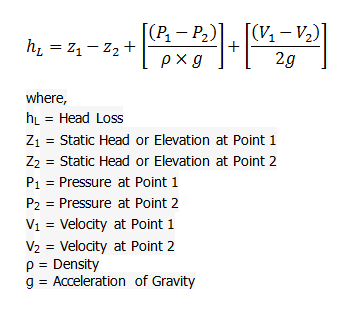# Bernoulli Theorem for Loss in Energy Head Calculator

This CalcTown calculator calculates the loss in Energy head for an inviscid fluid using Bernoulli Theorem. Bernoulli's principle states that for an inviscid flow of a non-conducting fluid, an increase in the speed of the fluid occurs simultaneously with a decrease in pressure or a decrease in the fluid's potential energy.

m
m/s
m/s
kg/m3
m/s2

#### Result

meters